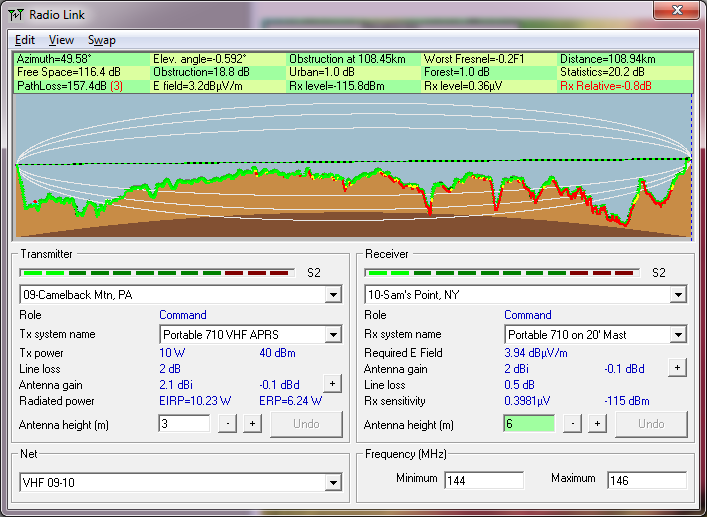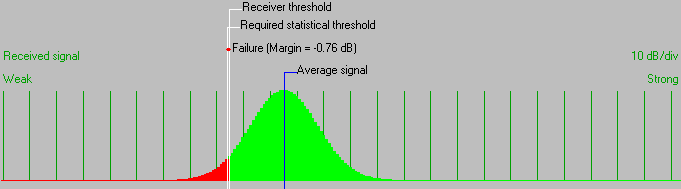• Distance between 09-Camelback Mtn, PA and 10-Sam's Point, NY is 108.9 km (67.7 miles)
• True North Azimuth = 49.58°, Magnetic North Azimuth = 61.85°, Elevation angle = -0.5924°
• Terrain elevation variation is 552.9 m
• Propagation mode is scatter, single obstruction, 0.2F1 at 108.4km
• Average frequency is 145.000 MHz
• Free Space = 116.4 dB, Obstruction = 18.8 dB, Urban = 1.0 dB, Forest = 1.0 dB, Statistics = 20.2 dB
• Total propagation loss is 157.4 dB (interference mode (optimistic))
• System gain from 09-Camelback Mtn, PA to 10-Sam's Point, NY is 156.6 dB
• System gain from 10-Sam's Point, NY to 09-Camelback Mtn, PA is 156.6 dB
• Worst reception is 0.8 dB below the required signal to meet 95.000% of situations
• Warning 3